# Selina Concise Solutions for Chapter 15 Linear Inequations (including Number Line) Class 8 ICSE Mathematics

##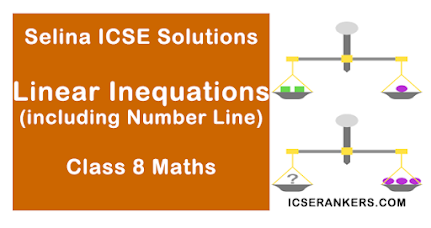### Exercise 15A

1. If the replacement set is the set of natural numbers, solve.
(i) x – 5 < 0
(ii) x + 1 < 7
(iii) 3x – 4 > 6
(iv) 4x + 1 > 17
Solution
(i) x – 5 < 0
⇒ x – 5 + 5 < 0 + 5 …(Adding 5)
⇒ x < 5
Required answer = {1, 2, 3, 4}

(ii) x + 1 ≤ 7
⇒ x + 1 – 1 ≤ 7 – 1 (Subtracting 1)
⇒ x ≤ 6
Required answer = {1, 2, 3, 4, 5, 6}

(iii) 3x – 4 > 6
3x – 4 + 4 > 6 + 4 (Adding 4)
⇒ 3x > 10
⇒ 3x/3 > 10/3 …(Dividing by 3)
⇒ x > 10/3
⇒ x > 3 1/3
Required answer = {4, 5, 6, …}

(iv) 4x + 1 ≥ 17
⇒ 4x + 1 – 1 ≥ 17 – 1 (Subtracting)
⇒ 4x ≥ 16
⇒ 4x/4  ≥ 16/4 (Dividing by 4)
⇒ x ≥ 4
Required answer = {4, 5, 6, …}

2. If the replacement set = {-6, -3, 0, 3, 6, 9}; find the truth set of the following:
(i) 2x – 1 > 9
(ii) 3x + 7 < 1
Solution
(i) 2x – 1 > 9
⇒ 2x – 1 + 1 > 9 + 1 (Adding 1)
⇒ 2x > 10
⇒ x > 5 (Dividing by 2)
⇒ x > 5

(ii) 3x + 7 ≤ 1
⇒ 3x + 7 – 7 ≤ 1 – 7 (Subtracting 7)
⇒ 3x ≤ – 6
⇒ x ≤ – 2

3. Solve 7 > 3x – 8; x ∈ N
Solution
7 > 3x – 8
⇒ 7 – 3x > 3x – 3x – 8 (Subtracting 3x)
⇒ 7 – 7 – 3x > 3x – 3x – 8 – 7 (Subtracting 7)
⇒ -3x > -15
⇒ x < 5 (Dividing by -3)
Required Answer = {1, 2, 3, 4}
Note : Division by negative number reverses the inequality.

4. -17 < 9y – 8 ; y ∈ Z
Solution
-17 < 9y – 8
⇒ -17 + 8 < 9y – 8 + 8 (Adding 8)
⇒ -9 < 9y
⇒ -1 < y (Dividing by 9)
Required number = {0, 1, 2, 3, 4, …}

5. Solve 9x – 7 ≤ 28 + 4x; x ∈ W
Solution
9x – 1 ≤ 28 + 4x
⇒ 9x – 4x – 7 ≤ 28 + 4x – 4x (Subtracting 4x)
⇒ 5x – 7 ≤ 28
⇒ 5x – 7 + 7 ≤ 28 + 7 (Adding 7)
⇒ 5x ≤ 35
⇒ x ≤ 7 (Dividing by 5)
Required answer = {0, 1, 2, 3, 4, 5, 6, 7}

6. Solve : 2/3x + 8 < 12 ; x ∈ W
Solution
2/3x + 8 < 12
⇒ 2/3x + 8 – 8 < 12 – 8
⇒ 2/3x < 4
⇒ 2/3x × 3/2 < 4 × 3/2  (Multiplying by 3/2)
⇒ x < 6
∴ Required answer = {0, 1, 2, 3, 4, 5}

7. Solve : -5(x + 4) > 30 ; x ∈ Z
Solution
-5 = (x + 4) > 30/-5  ...(Dividing by – 5)
Note: Division by a negative number reverses the equality
⇒ x + 4 < -6
⇒ x + 4 – 4 < - 6 – 4  (Subtracting 4)
⇒ x < -10
∴ Required Answer = {-11, -12, -13, …..}

8. Solve the inquation 8 – 2x > x – 5 ; x ∈ N.
Solution
8  - 2x ≥ x – 5 (x - 5 ; x ∈ N)
⇒ 8 + 5 ≥ 2x + x
⇒ 13 ≥ 3x
⇒ 3x  ≤ 13
⇒ x ≤ 13/3 = 4 1/3
x = 1, 2, 3, 4 (x ∈ N)
Solution set = {1, 2, 3, 4}

9. Solve the inequality 18 – 3 (2x – 5) > 12; x ∈ W.
Solution
18 – 3/(2x – 5) > 12; x ∈ W
⇒ 18 – 6x + 15 > 12
⇒ 3 – 6x > 12
Subtracting -3 from both side
-3 + 3 – 6x > 12 – 3
⇒-6x  > 15
⇒ 6x < -15 (Changing sign)
Dividing 6
x < -15/6
⇒ x < -2.5
But x ∈ W,  x = 0, 1, 2, 3
∴ Solution set  = {0, 1, 2, 3}

10. Solve : (2x + 1)/3 + 15 < 17 ; x ∈ W.
Solution
(2x + 1)/3 + 15 ≤ 17; x ∈ W
⇒ (2x + 1)/3 ≤ 17 – 15 = 2
⇒ 2x + 1 ≤ 6
⇒ 2x ≤ 5
⇒ x ≤ 5/2 = 2 ½
But x ∈ W
∴ x = 0, 1, 2
∴ Solution set is = {0, 1, 2}

11.  Solve : -3 + x < 2, x ∈ N
Solution
-3 + x < 2, x ∈ N
⇒ x < 2 – (-3)
⇒ x < 2 + 3
⇒ x < 5
∴ x = 1, 2, 3, 4    (∵  x ∈ N)
∴ Solution set = {1, 2, 3, 4}

12. Solve : 4x – 5 > 10 – x, x ∈ {0, 1, 2, 3, 4, 5, 6, 7}
Solution
4x – 5 > 10 – x, x ∈ N
⇒ 4x + x > 10 + 5
⇒ 5x > 15
⇒ x > 15/5 = 3
∴ x = 4, 5, 6, 7
Solution set = {4, 5, 6, 7}

13. Solve : 15 – 2(2x – 1) < 15, x ∈ Z.
Solution
15 – 2(2x – 1) < 15, x ∈ Z.
⇒ 15 – 4x + 2 < 15
⇒ 17 – 4x < 15
⇒ -4x < 15 – 17
⇒ -4x < -2
⇒ -4/-4x > -2/-4 = ½  (Dividing  by – 4)
∴ x = 1, 2, 3, 4, 5, ……
∴ Solution set = {1, 2, 3, 4, 5, ……}

14. Solve: (2x + 3)/5 > (4x – 1)/2, x ∈ W.
Solution
(2x + 3)/5 > (4x – 1)/2, x ∈ W
⇒ 2(2x + 3) > 5(4x – 1)
⇒ 4x + 6 > 20x – 5
⇒ 4x – 20x > - 5 – 6
⇒ -16x > -11
⇒ x < -11/-16  (Dividing by -16)
⇒ x < 11/16
∴ x = 0
∴ Solution set = {0}

### Exercise 15 B

Solve and graph the solution set on a number line:

1. x – 5 < -2 ; x ∈ N
Solution
x – 5 < -2
⇒ x – 5 + 5 < -2 + 5 (Adding 5 to both sides)
⇒ x < 3
∴ The required graph is:2. 3x – 1 > 5 ; x ∈ W
Solution
3x – 1 > 5
⇒ 3x – 1 +1 > 5 + 1  (Adding 1 to both sides)
⇒ 3x > 6
⇒ 3x/3 > 6/3  (Dividing both sides by 3)
⇒ x > 2
⇒ The required graph is :3. -3x + 12 < -15 ; x ∈ R.
Solution
-3x + 12 <  -15
⇒ -3x + 12 – 12 < -15 – 12  (Subtracting 12 from both sides)
⇒ -3x < - 27
⇒ -3x/-3 > -27/-3 (Dividing both sides by -3)
Note: Division by a negative number reverses the inequality.
⇒ x > 9
∴ The required graph is:4. 7 > 3x – 8; x ∈ W
Solution
7 ≥ 3x – 8
⇒ 7 + 8 ≥ 3x – 8 + 8  (Adding 8 to both sides)
⇒ 15 ≥ 3x
⇒ 15/3 ≥ 3x/3  (Diving both sides by 3)
⇒ 5 ≥ x
∴ The required graph is :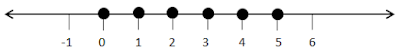5. 8x – 8 < – 24 ; x ∈ Z
Solution
8x – 8 ≤ -24
⇒ 8x – 8 + 8  ≤ -24 + 8  (Adding 8 to both sides)
⇒ 8x ≤ -16
⇒ 8x/8 ≤ -16/8  (Dividing both sides by 8)
⇒ x ≤ -2
∴ The required graph is :6. 8x – 9 > 35 – 3x; x ∈ N
Solution
8x – 9 ≥ 35 – 3x
⇒ 8x + 3x – 9 ≥ 35 – 3x + 3x  (Adding 3x to both sides)
⇒ 11x – 9 ≥ 35
⇒ 11x – 9 + 9 ≥ 35 + 9  (Adding 9 to both sides)
⇒ 11x ≥ 44
⇒ 11x/11 ≥ 44/11  (Dividing both sides by 11)
⇒ x ≥ 4
∴ The required graph is:7. 5x + 4 > 8x – 11; x ∈ Z
Solution
5x + 4 > 8x – 11
⇒ 5x – 5x + 4 > 8x – 5x – 11  (Subtracting from both sides)
⇒ 4 > 3x – 11
⇒ 4 + 11 > 3x – 11 + 11  (Adding 11 to both sides)
⇒ 15 > 3x
⇒ 15/3 > 3x/3  (Dividing both sides by 3)
⇒ 5 > x
∴ The required graph is :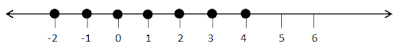8. 2x/5 + 1 < -3 ; x ∈ R
Solution
2x/5 + 1 < -3
⇒ 2x/5 + 1 – 1 < - 3 – 1 (Subtracting 1 from both sides)
⇒ 2x/5 < -4
⇒ 2x/5 × 5 <- 4 × 5  (Multiplying both sides by 5)
⇒ 2x < -20
⇒ 2x/2 < -20/2  (Dividing  both sides by 2)
⇒ x < -10
∴ The required  graph is:9. x/2 > -1 + 3x/4 ; x ∈ N
Solution
x/2 > -1 + 3x/4
⇒ x/2 ×4 > -1×4 + 3x/4 ×4  (Multiplying both sides by 4)
⇒ 2x > -4 = 3x
⇒ 2x – 2x > -4 + 3x- 2x  (Subtracting 2x  from both sides)
⇒ 0 > -4 + x
⇒ 0 + 4 > -4 + 4 + x (Adding 4 to both sides)
⇒ 4 > x
∴ The required  graph is: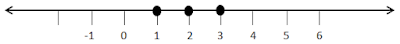10. 2/3x+ 5 ≤ ½ x + 6 ; x ∈ W
Solution
2/3x + 5 ≤ 1/2x + 6
⇒ 2/3x ×6 + 5 × 6 ≤ 1/2x ×6 + 6×6  (Multiplying both sides by 6)
⇒ 4x - 3x + 30 ≤ 3x – 3x + 36 (Subtracting 3x from both sides)
⇒  x + 30 ≤ 36
⇒ x + 30 – 30 ≤ 36 – 30  (Subtracting 30 from both sides)
x ≤ 6
∴ The required graph is :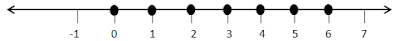11. Solve the inequation  5(x – 2) > 4 (x + 3) – 24 and represent its solution on a number line.
Given the replacement set is {-4, -3, -2, -1, 0, 1, 2, 3, 4}.
Solution
5(x – 2) > 4(x + 3) – 24
⇒ 5x – 10 > 4x +12 – 24
⇒ 5x – 4x > 10 – 12
⇒ x > -2
Since replacement set = {-4, -3, -2, -1, 0, 1, 2, 3, 4}
∴ Solution set = {-1, 0, 1, 2, 3, 4}
∴ Solution set on a number line is shown below.12. Solve  2/3(x – 1) + 4 < 10 and represent its solution on a number line.
Given replacement set is {-8, -6, -4, 3, 6, 8, 12}.
Solution
2/3(x – 1) + 4 < 10
⇒ 2/3(x – 1) < 10 – 4
⇒ 2/3(x – 1) < 6
⇒ 2(x – 1) < 6
⇒ x – 1 < 9
⇒ x + 1 + 1 < 9 + 1 (Adding 1 to both sides)
⇒ x < 10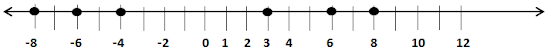Thus, x < 10
Since, replacement set = {-8, -6, -4, 3, 6, 8, 12}
Solution set = {-8, -6, -4, 3, 6, 8}

13. For each inequation, given below, represent the solution on a number line :
(i) 5/2 – 2x ≥ 1/2 ; x ∈ W
(ii) 3(2x – 1) ≥ 2(2x + 3), x ∈ Z
(iii) 2(4 – 3x) ≤ 4(x – 5), x ∈ W
(iv) 4(3x + 1) > 2(4x – 1), x is a negative integer
(v) (4 – x)/2 < 3, x ∈ R
(vi) -2(x + 8) ≤ 8, x ∈ R
Solution
(i) 5/2 – 2x ≥ ½, x ∈ W
⇒ -2x ≥ ½ - 5/2
⇒ -2x ≥ -4/2
⇒ -2x > - 2
⇒ x ≤ 1
∴ x = {0, 1}(ii) 3(2x – 1) ≥ 2(2x + 3), x ∈ Z
⇒ 6x – 3 ≥ 4x + 6 , x ∈ Z
⇒ 6x – 4x ≥ 6 + 3
⇒ 2x ≥ 9
⇒ x ≥ 9/2
⇒ x ≥ 4 ½
∴ x = {5, 6, 7, ….}(iii) 2(4 – 3x) ≤ 4(x – 5), x ∈ W
8 – 6x ≤ 4x – 20
⇒ -6x – 4x ≤ 4x – 20
⇒ -10x ≤ -28
⇒ 10x ≥ 28
⇒ x ≥ 28/10
⇒ x ≥ 2.8
∴ x = {3, 4, 5,….}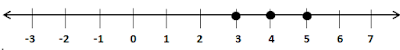(iv) 4(3x + 1) > 2(4x – 1), x is a negative integer
⇒ 12x + 4 > 8x – 2
⇒ 12x – 8x > -2 – 4
⇒ 4x > -6
⇒ x > -6/4
⇒ x > -1.5
∴ x = {1}(v) (4 – x)/2 < 3, x ∈ R
⇒ 4 – x < 6
⇒ -x < 6 – 4
⇒ -x < 2
⇒ x > - 2
∴ x > - 2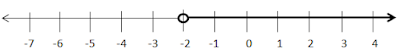(vi) -2(x + 8) ≤ 8, x ∈ R
-2x – 16 ≤ 18
⇒ -2x ≤ 8 + 16
⇒ -2x ≤ 24
⇒ x ≥ -24/2
x ≥ -12